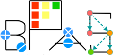Basic Polynomial Algebra Subprograms (BPAS)  v. 1.791
BPASGCDDomain< Derived > Class Template Referenceabstract

An abstract class defining the interface of a GCD domain. More...

`#include <BPASGCDDomain.hpp>`

Simplified semantic inheritance diagram for BPASGCDDomain< Derived >:Full inheritance diagram for BPASGCDDomain< Derived >:
[legend]

## Public Member Functions

virtual Derived gcd (const Derived &other) const =0
Get GCD of *this and other. More...

virtual Factors< Derived > squareFree () const =0
Compute squarefree factorization of *this. More...Public Member Functions inherited from BPASIntegralDomain< Derived >
virtual Derived operator/ (const Derived &d) const =0
Exact division. More...

virtual Derived & operator/= (const Derived &d)=0
Exact division assignment. More...Public Member Functions inherited from BPASRing< Derived >
virtual mpz_class getCharacteristic () const
The characteristic of this ring class.

virtual bool isZero () const =0
Determine if *this ring element is zero, that is the additive identity. More...

virtual void zero ()=0
Make *this ring element zero.

virtual bool isOne () const =0
Determine if *this ring element is one, that is the multiplication identity. More...

virtual void one ()=0
Make *this ring element one.

virtual Derived unitCanonical (Derived *u=NULL, Derived *v=NULL) const =0
Obtain the unit normal (a.k.a canonical associate) of an element. More...

virtual Derived & operator= (const Derived &)=0
Copy assignment.

virtual Derived operator+ (const Derived &) const =0

virtual Derived & operator+= (const Derived &)=0

virtual Derived operator- (const Derived &) const =0
Subtraction.

virtual Derived & operator-= (const Derived &)=0
Subtraction assignment.

virtual Derived operator- () const =0
Negation.

virtual Derived operator* (const Derived &) const =0
Multiplication.

virtual Derived & operator*= (const Derived &)=0
Multiplication assignment.

virtual Derived operator^ (long long int e) const =0
Exponentiation.

virtual Derived & operator^= (long long int e)=0
Exponentiation assignment.

virtual bool operator== (const Derived &) const =0
Equality test,. More...

virtual bool operator!= (const Derived &) const =0
Inequality test,. More...

virtual void print (std::ostream &ostream) const
Print the Ring element. More...

virtual std::string toString () const
Convert the Ring element to a string. More...Public Member Functions inherited from ExpressionTreeConvert
virtual ExpressionTree convertToExpressionTree () const =0
Convert this to an expression tree. More...

## Detailed Description

### template<class Derived> class BPASGCDDomain< Derived >

An abstract class defining the interface of a GCD domain.

## ◆ gcd()

template<class Derived>
 virtual Derived BPASGCDDomain< Derived >::gcd ( const Derived & other ) const
pure virtual

Get GCD of *this and other.

Parameters
 other the other element to get a gcd with.
Returns
the gcd.

## ◆ squareFree()

template<class Derived>
 virtual Factors BPASGCDDomain< Derived >::squareFree ( ) const
pure virtual

Compute squarefree factorization of *this.

Returns
the square free factorization as a Factors object.

The documentation for this class was generated from the following file: